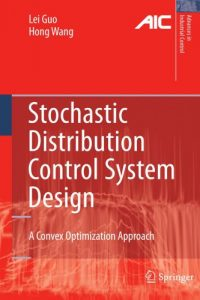اسم المؤلف
Lei Guo , Hong Wang
التاريخ
21 يونيو 2020
المشاهدات
195
التقييم(لا توجد تقييمات)Loading...

Stochastic Distribution Control System Design
A Convex Optimization Approach
Lei Guo , Hong Wang
Contents
1 Developments in Stochastic Distribution Control Systems . 1
1.1 Introduction . 1
1.1.1 Paper Web Formation Systems . 3
1.1.2 Flame Distribution Control 4
1.1.3 Challenging Issues . 6
1.2 Stochastic Distribution Control when Output PDFs are Measurable . 8
1.3 Stochastic Distribution Control when Output PDFs are
Unmeasurable . 11
1.4 Minimum Entropy Control . 12
1.5 Stochastic Distribution Filtering Design 13
1.6 Conclusions . 14
Part I Structural Controller Design for Stochastic Distribution Control
Systems
2 Proportional Integral Derivative Control for Continuous-time
Stochastic Systems . 17
2.1 Introduction . 17
2.2 Problem Formulation . 18
2.2.1 Model Representation 18
2.2.2 Pseudo-PID Controller Structure . 19
2.3 Pseudo-PID Controller Design 21
2.3.1 Solvability Condition . 21
2.3.2 Feasible Design Procedures 23
2.3.3 Robust Pseudo-PID Controller . 26
2.4 Simulations . 27
2.5 Conclusions . 29
3 Constrained Continuous-time Proportional Integral Derivative
Control Based on Convex Algorithms . 31
3.1 Introduction . 31
xiiiContents
3.2 Problem Formulation . 32
3.2.1 B-spline Expansion and Dynamic Weight Model 32
3.2.2 Generalized PID Controller Design . 34
3.3 Constrained PID Controller Design Based on LMIs 35
3.3.1 Peak-to-peak Performance Control 35
3.3.2 Peak-to-peak Tracking Performance 38
3.3.3 Peak-to-peak Constrained Tracking Control 40
3.4 Simulations . 41
3.5 Conclusions . 42
4 Constrained Discrete-time Proportional Integral Control Based on
Convex Algorithms . 45
4.1 Introduction . 45
4.2 Problem Formulation . 45
4.2.1 B-spline Expansion and Discrete-time Weight Dynamical
Model 45
4.2.2 Target Weight Model and Discrete-time PI Controller 47
4.3 Robust Constrained Tracking Control 48
4.3.1 Solvability for Peak-to-peak Performance 48
4.3.2 Peak-to-peak Tracking Performance 51
4.3.3 Constrained Peak-to-peak Tracking Control 53
4.4 Simulations . 54
4.5 Conclusions . 55
Part II Two-step Intelligent Optimization Modeling and Control for
Stochastic Distribution Control Systems
5 Adaptive Tracking Stochastic Distribution Control for Two-step
Neural Network Models . 63
5.1 Introduction . 63
5.2 Output PDF Model Using B-spline Neural Network 64
5.3 Dynamic Neural Network Identification 65
5.4 Adaptive Tracking Control for Weight Reference Model 68
5.5 Illustrative Examples 71
5.5.1 Example 1 71
5.5.2 Example 2 72
5.6 Conclusions . 76
6 Constrained Adaptive Proportional Integral Tracking Control for
Two-step Neural Network Models with Delays . 81
6.1 Introduction . 81
6.2 Output PDF Model Using B-spline Neural Network 82
6.3 Time Delay DNNs Identification 83
6.4 Constrained PI Tracking Control for Weight Vector 87
6.5 Illustrative Examples 91
6.5.1 Example 1 91
xivContents
6.5.2 Example 2 93
6.6 Conclusions . 97
7 Constrained Proportional Integral Tracking Control for
Takagi-Sugeno Fuzzy Model . 101
7.1 Introduction . 101
7.2 Problem Statement and Preliminaries 101
7.2.1 Output PDF Model Using B-spline Expansion 101
7.2.2 PI Controller Design Based on T-S Fuzzy Model 103
7.3 Main Results 104
7.3.1 Stability Analysis with L1 Measure Index 104
7.3.2 Peak-to-peak Tracking Performance 106
7.3.3 Constrained Peak-to-peak Tracking Control 108
7.4 An Illustrative Example . 109
7.5 Conclusions . 110
Part III Statistical Tracking Control – Driven by Output Statistical
Information Set
8 Multiple-objective Statistical Tracking Control Based on Linear
Matrix Inequalities . 115
8.1 Introduction . 115
8.2 Problem Formulation and Preliminaries 115
8.3 Formulation for the H2/H? Tracking Problem 117
8.4 Illustrative Examples 122
8.5 Conclusions . 125
9 Adaptive Statistical Tracking Control Based on Two-step Neural
Networks with Time Delays . 129
9.1 Introduction . 129
9.2 Problem Formulation and Preliminaries 129
9.3 Time Delay DNNs Identification 130
9.4 Variable Structure Tracking Control for Weight Vectors . 132
9.5 An Illustrative Example . 136
9.6 Conclusions . 138
Part IV Fault Detection and Diagnosis for Stochastic Distribution Control
Systems
10 Optimal Continuous-time Fault Detection Filtering 145
10.1 Introduction . 145
10.2 Problem Formulation . 146
10.3 Main Result . 147
10.4 Numerical Examples 152
10.5 Conclusions . 155
xvxvi Contents
11 Optimal Discrete-time Fault Detection and Diagnosis Filtering 157
11.1 Introduction . 157
11.2 Formulation of the FDD Problem with Guaranteed Cost Performance158
11.3 The Optimal Fault Detection Filter Design . 160
11.4 Fault Diagnosis Filter Design . 162
11.5 Simulation Examples . 165
11.6 Conclusions . 166
Part V Conclusions
12 Summary and Potential Applications . 173
12.1 Summary . 173
12.2 Potential Applications of PDF Control . 174
12.2.1 PDF Control in Data-driven Modeling . 174
12.2.2 PDF Control in Data Dimension Reduction 175
12.2.3 PDF Control in Multi-scale Plant-wide Modeling for Fault
Diagnosis and Fault Tolerant Control 175
References . 179
Index . 193
كلمة سر فك الضغط : books-world.net